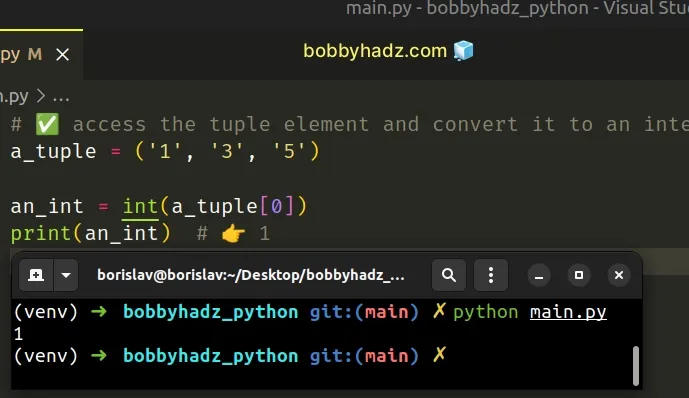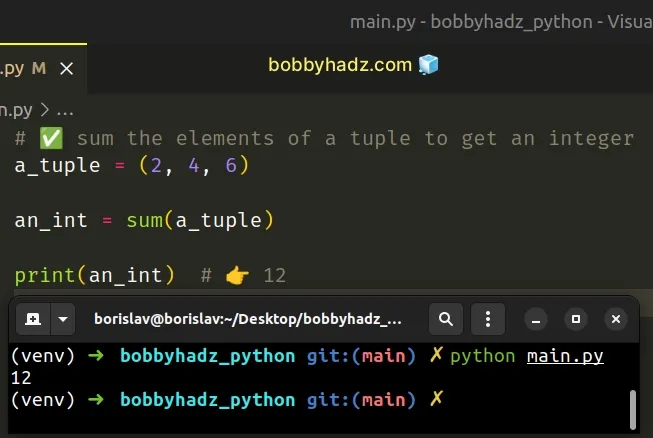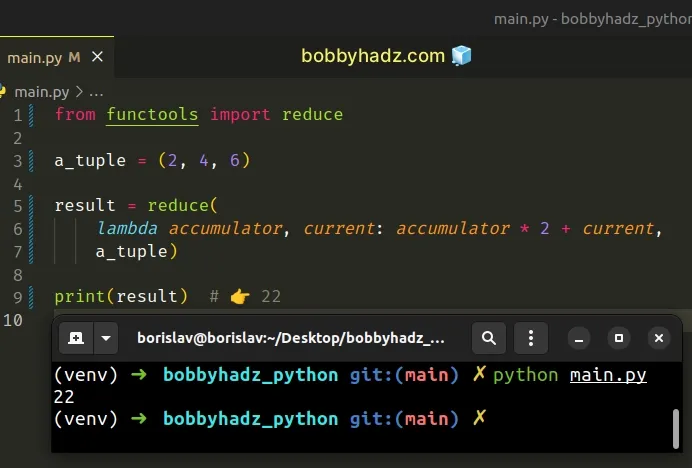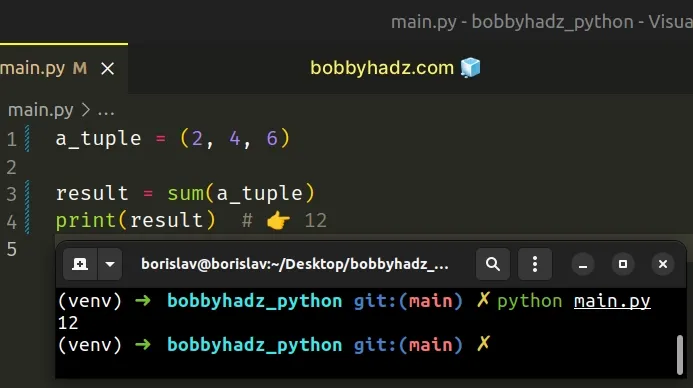# How to convert a Tuple to an Integer in PythonLast updated: Feb 19, 2023
5 min## #Convert a tuple to an integer in Python

There are multiple ways to convert a tuple to an integer:

1. Access a tuple element at its index and convert it to an int, e.g. `int(my_tuple)`.
2. Sum or multiply the elements of the tuple.
3. Convert a tuple of strings to a tuple of integers.
main.py
```Copied!```# ✅ access the tuple element and convert it to an integer
a_tuple = ('1', '3', '5')

an_int = int(a_tuple)
print(an_int)  # 👉️ 1
``````If you need to sum the elements of a tuple to get an integer, use the following code sample instead.

main.py
```Copied!```# ✅ sum the elements of a tuple to get an integer
a_tuple = (2, 4, 6)

an_int = sum(a_tuple)

print(an_int)  # 👉️ 12
``````The first example accesses the tuple element at a specific index and uses the `int()` class to convert it to an integer.

main.py
```Copied!```a_tuple = ('1', '3', '5')

an_int = int(a_tuple)
print(an_int)  # 👉️ 1
``````
Python indexes are zero-based, so the first element in a tuple has an index of `0`, the second an index of `1`, etc.

When the index starts with a minus, we start counting backward from the end of the tuple.

For example, the index `-1` gives us access to the last element, `-2` to the second-last, etc.

main.py
```Copied!```my_tuple_1 = ('1', '3', '5')

my_integer = int(my_tuple_1[-1])
print(my_integer)  # 👉️ 5
``````

You only have to use the `int()` class if your tuple doesn't store integers.

Otherwise, directly access the tuple element at its index.

main.py
```Copied!```my_tuple_1 = (1, 3, 5)

my_integer = my_tuple_1
print(my_integer)  # 👉️ 3
``````

## #Convert a tuple to an integer using reduce()

You can also use the `reduce()` function from the `functools` module to convert a tuple to an integer.

main.py
```Copied!```from functools import reduce

a_tuple = (2, 4, 6)

result = reduce(
lambda accumulator, current: accumulator * 2 + current,
a_tuple)

print(result)  # 👉️ 22
``````The reduce function takes the following 3 parameters:

NameDescription
functionA function that takes 2 parameters - the accumulated value and a value from the iterable.
iterableEach element in the iterable will get passed as an argument to the function.
initializerAn optional initializer value that is placed before the items of the iterable in the calculation.

The lambda function we passed to the `reduce()` gets called with the accumulated value and the current tuple element.

The code sample multiplies the accumulator by two and adds the current tuple element to the result.

main.py
```Copied!```from functools import reduce

a_tuple = (2, 4, 6)

result = reduce(
lambda accumulator, current: accumulator * 2 + current,
a_tuple)

print(result)  # 👉️ 22
``````

## #Convert a tuple to an integer using join()

You can also use the str.join() method to convert a tuple to an integer.

main.py
```Copied!```a_tuple = (2, 4, 6)

result = int(''.join(map(str, a_tuple)))

print(result) # 👉️ 246
``````The map() function takes a function and an iterable as arguments and calls the function with each item of the iterable.

We passed the str() class to the `map()` function to convert each element of the tuple to a string.

The next step is to use the `str.join()` method to join the tuple into a string.

main.py
```Copied!```a_tuple = (2, 4, 6)

print(''.join(map(str, a_tuple))) # 👉️ "246"
``````

The last step is to convert the string to an integer with the `int()` class.

## #Convert a tuple to an integer with the sum() function

You can also convert a tuple to an integer by using the `sum()` function.

main.py
```Copied!```a_tuple = (2, 4, 6)

result = sum(a_tuple)
print(result)  # 👉️ 12
``````The sum function takes an iterable, sums its items from left to right and returns the total.

main.py
```Copied!```my_list = [10, 20, 30]

print(sum(my_list))  # 👉️ 60
``````

## #Convert a tuple to an integer using a `for` loop

You can also use a for loop to convert a tuple to an integer.

main.py
```Copied!```a_tuple = (2, 4, 6)

result = ''

for number in a_tuple:
result += str(number)

result = int(result)

print(result) # 👉️ 246
``````

We declared a new variable that stores an empty string and used a `for` loop to iterate over the tuple.

On each iteration, we convert the current tuple element to a string and append it to the result.

The last step is to convert the `result` string to an integer with the `int()` class.

## #Convert a tuple of strings to a tuple of integers in Python

To convert a tuple of strings to a tuple of integers:

1. Use a generator expression to iterate over the tuple.
2. Convert each tuple item to an integer by passing them to the `int()` class.
3. Use the `tuple()` class to convert the generator object to a tuple.
main.py
```Copied!```tuple_of_strings = ('10', '20', '30', '40')

tuple_of_integers = tuple(int(item) for item in tuple_of_strings)

# 👇️ (10, 20, 30, 40)
print(tuple_of_integers)
``````

We used a generator expression to iterate over the tuple of strings.

Generator expressions are used to perform some operation for every element or select a subset of elements that meet a condition.

On each iteration, we pass the tuple element to the `int()` class to convert it to an integer.

The int class returns an integer object constructed from the provided number or string argument.

Alternatively, you can use the `map()` function.

## #Convert a tuple of strings to a tuple of integers using `map()`

This is a three-step process:

1. Pass the `int()` class to the `map()` function.
2. The `map()` function will pass each item of the tuple to the `int()` class.
3. The new tuple will only contain integer values.
main.py
```Copied!```tuple_of_strings = ('10', '20', '30', '40')

tuple_of_integers = tuple(map(int, tuple_of_strings))

# 👇️ (10, 20, 30, 40)
print(tuple_of_integers)
``````

The map() function takes a function and an iterable as arguments and calls the function with each item of the iterable.

This approach is a bit more implicit than using a generator expression.

The `map()` function returns a `map` object, so we had to use the `tuple()` class to convert the `map` object to a tuple.

Tuples are very similar to lists, but implement fewer built-in methods and are immutable (cannot be changed).

Since tuples cannot be changed, the only way to convert a tuple of strings to a tuple of integers is to create a new tuple that contains integer values.

Which approach you pick is a matter of personal preference. I'd go with the generator expression as I find it more direct and more explicit.